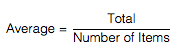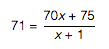# MCQ Quantitative Comparison - Quantitative Comparison

The CAT Online Free Test Prep MCQs Quantitative Comparison - Quantitative Comparison

### Quantitative Comparison: Quantitative Comparison

#### Question:

 Column A Column B The average (arithmetic mean) high temperature for x days is 70 degrees. The addition of one day with a high temperature of 75 degrees increases the average to 71 degrees. x 5
Option A:      Quantity A is greater.
Option B:      Quantity B is greater.
Option C:      The two quantities are equal.
Option D:      The relationship cannot be determined from the information given.

##### Explanation

If the average high temperature for x days is 70 degrees, then the sum of those x high temperatures is 70x. The sum of the high temperatures, including the additional day that has a temperature of 75 degrees is, therefore, 70x + 75. Next, use the average formula to find the value of x:In this formula, 71 is the average, 70x + 75 is the total, and there are x + 1 days. Substituting this information into the formula gives:To solve, cross-multiply to get 71x + 71 = 70x + 75. Next, simplify to find that x = 4. Therefore, Quantity B is greater. The correct answer is (B).

 1 of 10

### How to Solve Quantitative Comparison

For Quantitative Comparison question first understand the statement and the question stem. Try to solve and click the corresponding answer option. The drop down will appear to show the correct answer and explanation.